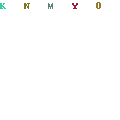# How To Calculate Concrete

Remember: 1 Cubic Yard of Concrete = 3′ x 3′ x 3′ = 27 cubic feet = 46,656 cubic inchesThis is an exercise aimed at a real world example of how to calculate concrete yardage needed to order for your slab placement. The given’s for this exercise in how to calculate concrete are as follows:
Slab Depth = 4″ (Four Inches)
Beam Width = 12″ (Twelve Inches)
Beam Depth = 32″ (Thirty-Two Inches) from Top of Form Board
Brick Ledge Width – 5 1/2″ (Five and a half inches) – Brick ledge with does not actually matter for the calculation
Garage floor and entry porch dropped approximately 4 inches from surface of slab.

Solution on How to Calculate Concrete for Above Example
Calculating Material Needed for 4 inch Slab (Large Section)
415.5″ x 527″ x 4″ = 875,874″/46656″ (1 cubic yard) = 18.77 Yards of Concrete.
Calculating Concrete Material Needed for 4 inch Slab (Small Section)
245″ x 106″ x 4″ = 103,880″/46656″ (1 cubic yard) = 2.23 Yards of Concrete.
Solution
Total Concrete in Beams = 33.25 Cubic Yards of Concrete
4″ Slab (Large) = 18.77 Cubic Yards of Concrete
4″ Slab (Small) = 2.23 Cubic yards of concrete
Total = 54.25 Cubic Yards of Concrete